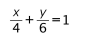# IMAT 2016 Q56 [Centre of Circle]

The straight­-line graph given by the equationintersects the x-­axis at A (a,0) and the y­axis at B (0,b).

A circle passes through A and B and has a diameter AB.

What are the coordinates of the centre of the circle?

A. (2, 3)
B. (3, 2)
C. (6, 4)
D. (4, 6)
E. (0, 0)

First of all, we should express the straight line as y=f(x)

\frac{x}{4} + \frac{y}{6} = 1 \to \frac{y}{6} = 1 -\frac{x}{4} \to y= 6 - 6\frac{x}{4} \to y= 6-\frac{3}{2}x

Now let’s draw that:

tip: To draw straight-line graph the fastest, find the values for x=0 and y=0 and draw a line through these dots

Looking at the graph, we can see that the line intersects with the x-axis at A(4,0) and the y-axis at B(0,6).

If the diameter of the circle is AB, that means that the centar of the circle is in the middle of AB, meaning, it’s coordinates must be (\frac{a}{2},\frac{b}{2}) =(\frac{4}{2},\frac{6}{2}) = (2,3)

So the correct answer is (A) (2,3)